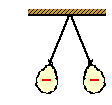# Electricity: Static Electricity

## Static Electricity: Audio Guided Solution

#### Problem 23:Two identical balloons are inflated and charged in the same manner. They are tied by threads and hung from the same pivot point on the ceiling. The balloons hang down, with the threads making an angle of 14° with each other and the balloons being separated by a distance of 58 cm (center-to-center). Each balloon has a charge of -3.5x10-7 C. Draw a free-body diagram for the balloons and consider them to act as point objects.
a. Calculate the force of electrical repulsion between the balloons.
b. What is the horizontal component of force in the thread that supports either one of the balloons?
c. What is the vertical component of force in the thread that supports either one of the balloons?
d. What is the mass of either one of the balloons?

## Audio Guided Solution

Click to show or hide the answer!

## Habits of an Effective Problem Solver

• Read the problem carefully and develop a mental picture of the physical situation. If necessary, sketch a simple diagram of the physical situation to help you visualize it.
• Identify the known and unknown quantities; record them in an organized manner. A diagram is a great place to record such information. Equate given values to the symbols used to represent the corresponding quantity - e.g., Q1 = 2.4 μC; Q2 = 3.8 μC; d = 1.8 m; Felect = ???.
• Use physics formulas and conceptual reasoning to plot a strategy for solving for the unknown quantity.
• Identify the appropriate formula(s) to use.
• Perform substitutions and algebraic manipulations in order to solve for the unknown quantity.